Published © CC BY-SA

# Stereo Vision and LiDAR Powered Donkey Car

Donkey Car featuring the Ultra96 board, a Raspberry Pi, FPGA accelerated stereo vision, MIPI CSI-2 image acquisition, a LiDAR sensor and AI.

AdvancedFull instructions provided3 days19,174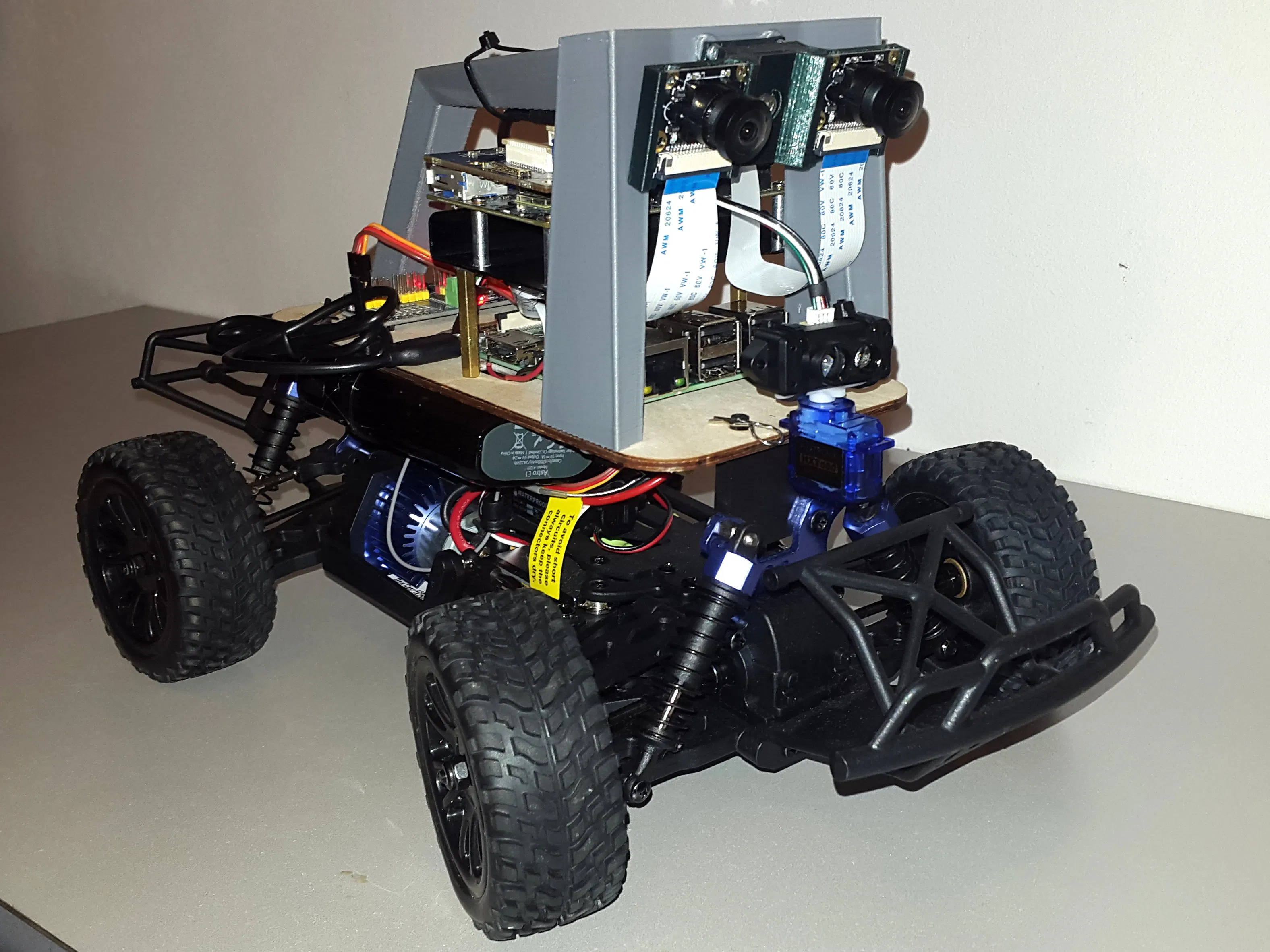## Things used in this project

### Hardware components

 RC Car
×1
 Donkey Car Kit
×1Raspberry Pi 3 Model B
×1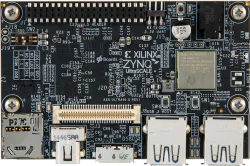Avnet Ultra96-V1
×1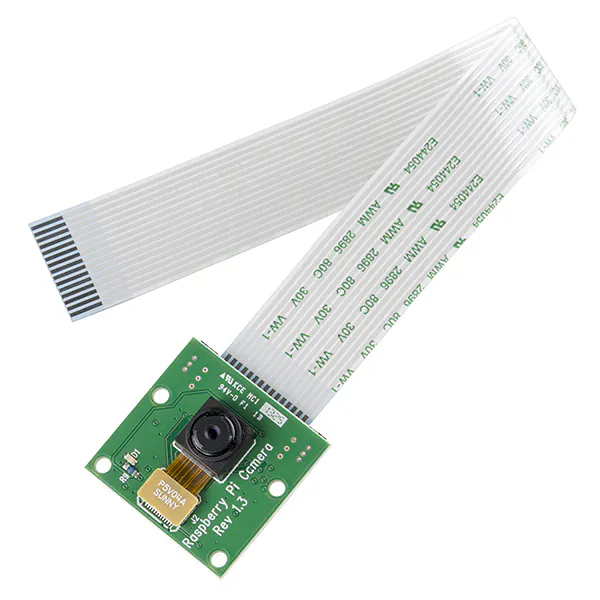Raspberry Pi Camera Module Preferably the night vision, wide view version. One camera module is included in the Donkey Car Kit.
×2
 TFMini LiDAR sensor
×1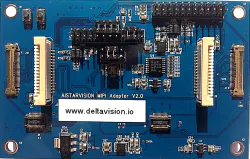96Boards DUAL MIPI Adapter Mezzanine - AiStarVision
×1

### Software apps and online services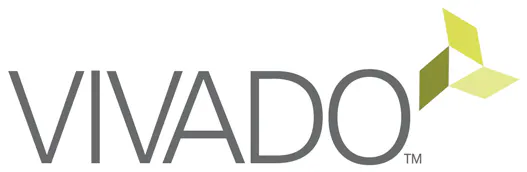Xilinx Vivado Design Suite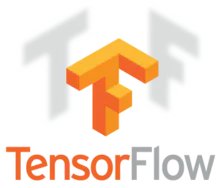TensorFlow
 Xilinx SDK
 Xilinx SDx (SDSoC development)
 Xilinx reVision (xfOpenCV)OpenCV

### Hand tools and fabrication machines3D Printer (generic)

Read more

## Schematics

### Schematics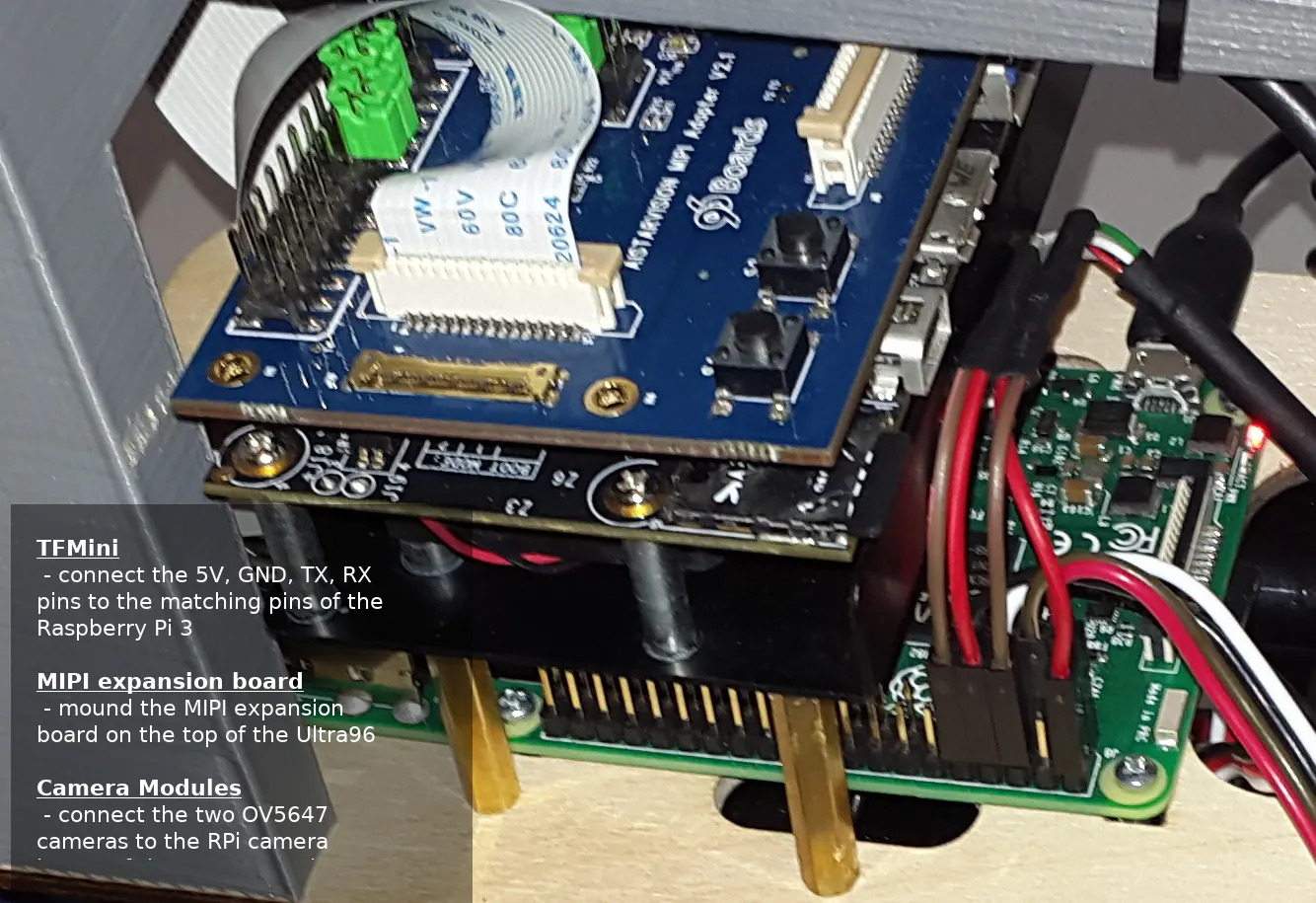### MIPI Adapter I2C Connections

Jumpers needed to connect the 2 camera's I2C interfaces to the Ultra96's I2C2, and I2C3 interfaces (red, orange) and camera GPIO pins (blue).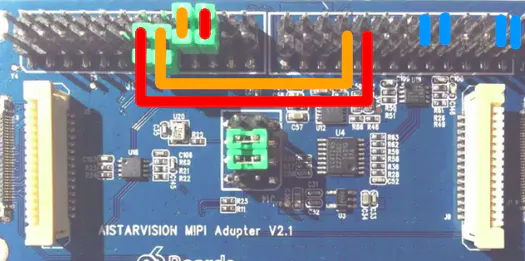## Code

### Vivado Project for Ultra96 with 2 x MIPI CSI-2 VIdeo Pipelines

Verilog
Vivado Project archive with 2 x MIPI CSI-2 VIdeo Pipelines.
The PFM properties are so the project is ready for DSA generation.
`No preview (download only).`

### PetaLinux Ultra96 2x MIPI CSI project-spec folder

SH
Contains the kernel patches (OV5647 and Xilinx driver) and device tree nodes.
`No preview (download only).`

### Donkey Car - updated manage.py

Python
```#!/usr/bin/env python3
"""
Scripts to drive a donkey 2 car and train a model for it.

Usage:
manage.py (drive) [--model=<model>] [--js] [--chaos]
manage.py (train) [--tub=<tub1,tub2,..tubn>]  (--model=<model>) [--base_model=<base_model>] [--no_cache]

Options:
-h --help        Show this screen.
--tub TUBPATHS   List of paths to tubs. Comma separated. Use quotes to use wildcards. ie "~/tubs/*"
--js             Use physical joystick.
--chaos          Add periodic random steering when manually driving
"""
import os
from docopt import docopt

import donkeycar as dk

#import parts
from donkeycar.parts.camera import PiCamera
from donkeycar.parts.transform import Lambda
from donkeycar.parts.keras import KerasCategorical
from donkeycar.parts.actuator import PCA9685, PWMSteering, PWMThrottle
from donkeycar.parts.datastore import TubGroup, TubWriter
from donkeycar.parts.controller import LocalWebController, JoystickController
from donkeycar.parts.clock import Timestamp

from lidar import TFMiniLidar

def drive(cfg, model_path=None, use_joystick=False, use_chaos=False):
"""
Construct a working robotic vehicle from many parts.
Each part runs as a job in the Vehicle loop, calling either
it's run or run_threaded method depending on the constructor flag `threaded`.
All parts are updated one after another at the framerate given in
cfg.DRIVE_LOOP_HZ assuming each part finishes processing in a timely manner.
Parts may have named outputs and inputs. The framework handles passing named outputs
to parts requesting the same named input.
"""

V = dk.vehicle.Vehicle()

clock = Timestamp()
V.add(clock, outputs='timestamp')

cam = PiCamera(resolution=cfg.CAMERA_RESOLUTION)
V.add(cam, outputs=['cam/image_array'], threaded=True)

lidar = TFMiniLidar()
V.add(lidar, outputs=['lidar/dist_array'], threaded=True)

if use_joystick or cfg.USE_JOYSTICK_AS_DEFAULT:
ctr = JoystickController(max_throttle=cfg.JOYSTICK_MAX_THROTTLE,
steering_scale=cfg.JOYSTICK_STEERING_SCALE,
auto_record_on_throttle=cfg.AUTO_RECORD_ON_THROTTLE)
else:
# This web controller will create a web server that is capable
# of managing steering, throttle, and modes, and more.
ctr = LocalWebController(use_chaos=use_chaos)

V.add(ctr,
inputs=['cam/image_array', 'lidar/dist_array'],
outputs=['user/angle', 'user/throttle', 'user/mode', 'recording'],
threaded=True)

# See if we should even run the pilot module.
# This is only needed because the part run_condition only accepts boolean
def pilot_condition(mode):
if mode == 'user':
return False
else:
return True

pilot_condition_part = Lambda(pilot_condition)
V.add(pilot_condition_part, inputs=['user/mode'],
outputs=['run_pilot'])

# Run the pilot if the mode is not user.
kl = KerasCategorical()
if model_path:
kl.load(model_path)

V.add(kl, inputs=['cam/image_array', 'lidar/dist_array'],
outputs=['pilot/angle', 'pilot/throttle'],
run_condition='run_pilot')

# Choose what inputs should change the car.
def drive_mode(mode,
user_angle, user_throttle,
pilot_angle, pilot_throttle):
if mode == 'user':
return user_angle, user_throttle

elif mode == 'local_angle':
return pilot_angle, user_throttle

else:
return pilot_angle, pilot_throttle

drive_mode_part = Lambda(drive_mode)
V.add(drive_mode_part,
inputs=['user/mode', 'user/angle', 'user/throttle',
'pilot/angle', 'pilot/throttle'],
outputs=['angle', 'throttle'])

steering_controller = PCA9685(cfg.STEERING_CHANNEL)
steering = PWMSteering(controller=steering_controller,
left_pulse=cfg.STEERING_LEFT_PWM,
right_pulse=cfg.STEERING_RIGHT_PWM)

throttle_controller = PCA9685(cfg.THROTTLE_CHANNEL)
throttle = PWMThrottle(controller=throttle_controller,
max_pulse=cfg.THROTTLE_FORWARD_PWM,
zero_pulse=cfg.THROTTLE_STOPPED_PWM,
min_pulse=cfg.THROTTLE_REVERSE_PWM)

V.add(steering, inputs=['angle'])
V.add(throttle, inputs=['throttle'])

# add tub to save data
inputs = ['cam/image_array', 'lidar/dist_array', 'user/angle', 'user/throttle', 'user/mode', 'timestamp']
types = ['image_array', 'ndarray', 'float', 'float',  'str', 'str']

#multiple tubs
#th = TubHandler(path=cfg.DATA_PATH)
#tub = th.new_tub_writer(inputs=inputs, types=types)

# single tub
tub = TubWriter(path=cfg.TUB_PATH, inputs=inputs, types=types)
V.add(tub, inputs=inputs, run_condition='recording')

# run the vehicle
V.start(rate_hz=cfg.DRIVE_LOOP_HZ,
max_loop_count=cfg.MAX_LOOPS)

def train(cfg, tub_names, new_model_path, base_model_path=None ):
"""
use the specified data in tub_names to train an artifical neural network
saves the output trained model as model_name
"""
X_keys = ['cam/image_array', 'lidar/dist_array']
y_keys = ['user/angle', 'user/throttle']
def train_record_transform(record):
""" convert categorical steering to linear and apply image augmentations """
record['user/angle'] = dk.util.data.linear_bin(record['user/angle'])
# TODO add augmentation that doesn't use opencv
return record

def val_record_transform(record):
""" convert categorical steering to linear """
record['user/angle'] = dk.util.data.linear_bin(record['user/angle'])
return record

new_model_path = os.path.expanduser(new_model_path)

kl = KerasCategorical()
if base_model_path is not None:
base_model_path = os.path.expanduser(base_model_path)
kl.load(base_model_path)

print('tub_names', tub_names)
if not tub_names:
tub_names = os.path.join(cfg.DATA_PATH, '*')
tubgroup = TubGroup(tub_names)
train_gen, val_gen = tubgroup.get_train_val_gen(X_keys, y_keys,
train_record_transform=train_record_transform,
val_record_transform=val_record_transform,
batch_size=cfg.BATCH_SIZE,
train_frac=cfg.TRAIN_TEST_SPLIT)

total_records = len(tubgroup.df)
total_train = int(total_records * cfg.TRAIN_TEST_SPLIT)
total_val = total_records - total_train
print('train: %d, validation: %d' % (total_train, total_val))
steps_per_epoch = total_train // cfg.BATCH_SIZE
print('steps_per_epoch', steps_per_epoch)

kl.train(train_gen,
val_gen,
saved_model_path=new_model_path,
steps=steps_per_epoch,
train_split=cfg.TRAIN_TEST_SPLIT)

if __name__ == '__main__':
args = docopt(__doc__)
cfg = dk.load_config()

if args['drive']:
drive(cfg, model_path = args['--model'], use_joystick=args['--js'], use_chaos=args['--chaos'])

elif args['train']:
tub = args['--tub']
new_model_path = args['--model']
base_model_path = args['--base_model']
cache = not args['--no_cache']
train(cfg, tub, new_model_path, base_model_path)
```

### Donkey Car - lidar.py - sensor part

Python
TFMiniLidar Vechicle Part
```import serial
import time
import numpy as np
import time

class TFMiniLidar:
def __init__(self):
self.ser = serial.Serial('/dev/ttyS0',115200,timeout = 1)

self.ser.write(bytes(b'B'))
self.ser.write(bytes(b'W'))
self.ser.write(bytes(2))
self.ser.write(bytes(0))
self.ser.write(bytes(0))
self.ser.write(bytes(0))
self.ser.write(bytes(1))
self.ser.write(bytes(6))

self.resolution = 90;

self.frame = np.zeros(self.resolution)

import Adafruit_PCA9685
self.pwm = Adafruit_PCA9685.PCA9685()
self.pwm.set_pwm_freq(60)
self.channel = 15

self.running = True

self.servo_min = 140
self.servo_max = 590

self.dir_range = range(0, self.resolution)
self.read_one_frame()

# Return Frame
def run_threaded(self):
return np.copy(self.frame)

def shutdown(self):
self.running = False

# Run
def run(self):
self.update()

def update(self):
while (self.running):
self.read_one_frame();

def read_one_frame(self):
for i in self.dir_range:
pulse = self.servo_min + (self.servo_max - self.servo_min) * i / self.resolution
self.pwm.set_pwm(self.channel, 0, int(round(pulse)))
#time.sleep(.0015)
self.frame[i] = self.read_one()

self.dir_range = list(reversed(self.dir_range))

def read_one(self):
#while (self.ser.in_waiting >= 9):
if ((b'Y' == self.ser.read()) and ( b'Y' == self.ser.read())):
Dist_L = self.ser.read()
Dist_H = self.ser.read()
Dist_Total = (ord(Dist_H) * 256) + (ord(Dist_L))
for i in range (0,5):
self.ser.read()

#print(Dist_Total)
return Dist_Total

if __name__ == "__main__":
lidar = TFMiniLidar()
print(lidar.run_threaded())
lidar.read_one_frame()
lidar.read_one_frame()
```

### updated donkeycar package

Python
/home/pi/env/lib/python3.5/site-packages/
`No preview (download only).`

### Stereo Calibration (FishEye cameras)

C/C++
Mainly inspired from: github.com/sourishg/fisheye-stereo-calibration
`No preview (download only).`

## Credits

### Attila Tőkés

32 projects • 172 followers
Software Engineer experimenting with hardware projects involving IoT, Computer Vision, ML & AI, FPGA, Crypto and other related technologies.